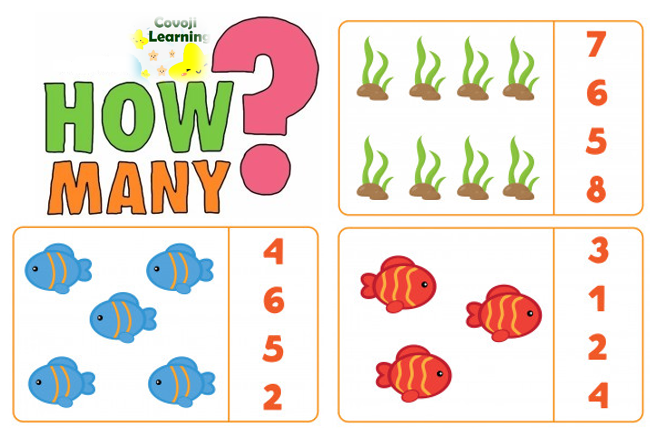A cardinal number is a number used in counting such as 1, 2, 3, 4, 5……

Cardinal numbers tell us “How many?”On counting, we get 3 Rilakkumas.

### Is zero a cardinal number?

No, zero (0) is not a cardinal number. This is because cardinal numbers tell us about quantity. Cardinal numbers start from “1” and do not have decimals, fractions or negative numbers.

Cardinal numbers are used to answer questions such as “How many children are on the playground?” or  “How many apples in the basket?”

### Count them!Learning to count is fun with Pick The Most. Check it out.

Categories: Math Zone